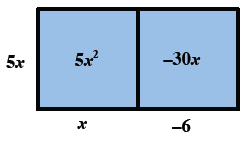### Home > GB8I > Chapter 4 Unit 5 > Lesson INT1: 4.1.2 > Problem4-22

4-22.

Rewrite each of the following products as a sum by using the Distributive Property. Homework Help ✎

1. $5x(x-6)$

• Using the Distributive Property, distribute the $5x$ over the two terms in the parentheses.

$5x(x) − 5x(6)$

You can also create a generic rectangle like the one below.$5x^2 − 30x$

1. $-9y(6-3y)$

• See the help for part (a).